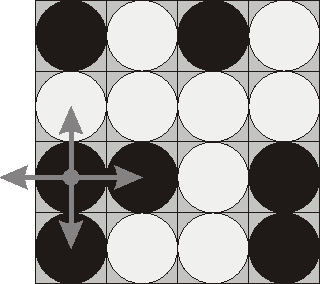Principle Researcher, AI & Software Engineering

## 题意简述

4*4的格子放有黑白两面的棋子16个，翻转任一棋子会使其上下左右棋子一同翻转。给定初始状态，求达到所有棋子全黑或全白的最少翻转次数。如果无解，输出Impossible。POJ1753附图

## 算法分析

1、翻转棋盘上任意个棋子，棋盘达到的状态与各棋子被翻转的先后顺序无关。

2、对每个棋子进行2次及以上的翻转没有意义。∵ 偶数次翻转结果与不翻转相同，奇数次翻转结果与翻转1次相同。

——————————————————————分割线——————————————————————

DFS。

`dfs(array,position,step,answer)`四个形参，array传递数组，position传递当前位置，step传递深度（也即翻转个数），ans传递当前最优答案。

`dfs(array,position+1,step+1,answer)`

`dfs(array,position+1,step,answer)`

Problem Status: AC。时间94ms，内存360k

——————————————————————分割线——————————————————————

TIPS:

WA了好几次。有几个小细节要注意：

1、翻转时，如果遇到行末则不翻转下一个，遇到行首则不翻转上一个。可以通过mod 4的值来判断。

2、DFS时，需先判断是否满足条件（全黑或全白）再判断位置是否越界（position<16）。因为有可能所有棋子都需要翻转，由于判断本次翻转是否成功是在下一层递归中，所以即使position越界也需先验证一次上次翻转后是否达到所需状态。

——————————————————————分割线——————————————————————

1、一维01数组可以使用一个int类型变量替代。2个字节16位就可以表示了。翻转操作采用位运算实现。（这个还没试过，据说时间达到16ms，以后有机会试下。）

2、网上的解题报告说据说有一种枚举可以达到时间0ms？还没找到具体算法。要去膜拜下。

## 程序样例

```#include<stdio.h>

void change(int s[], int pos) {
if (s[pos] == 0) s[pos] = 1;
else s[pos] = 0;
if ((pos + 1 < 16) && (pos % 4 != 3))
if (s[pos + 1] == 0) s[pos + 1] = 1;
else s[pos + 1] = 0;
if ((pos - 1 >= 0) && (pos % 4 != 0))
if (s[pos - 1] == 0) s[pos - 1] = 1;
else s[pos - 1] = 0;
if (pos + 4 < 16)
if (s[pos + 4] == 0) s[pos + 4] = 1;
else s[pos + 4] = 0;
if (pos - 4 >= 0)
if (s[pos - 4] == 0) s[pos - 4] = 1;
else s[pos - 4] = 0;
}

int finish(int s[]) {
int i;
for (i = 1; i < 16; i++)
if (s[i] != s) return 0;
return 1;
}

void
try (int s[], int pos, int step, int * ans) {
if (finish(s)) {
if (step < * ans) * ans = step;
return;
}
if (pos > 15) return;
change(s, pos);
try (s, pos + 1, step + 1, ans);
change(s, pos);
try (s, pos + 1, step, ans);
}

int main() {
int s;
int i, j, ans = 17;
char t;
for (i = 0; i < 4; i++) {
scanf("%s", t);
for (j = 0; j < 4; j++)
if (t[j] == 'b') s[i * 4 + j] = 1;
else s[i * 4 + j] = 0;
}
try (s, 0, 0, & ans);
if (ans == 17) printf("Impossible");
else printf("%d", ans);
system("pause");
return 0;
}```